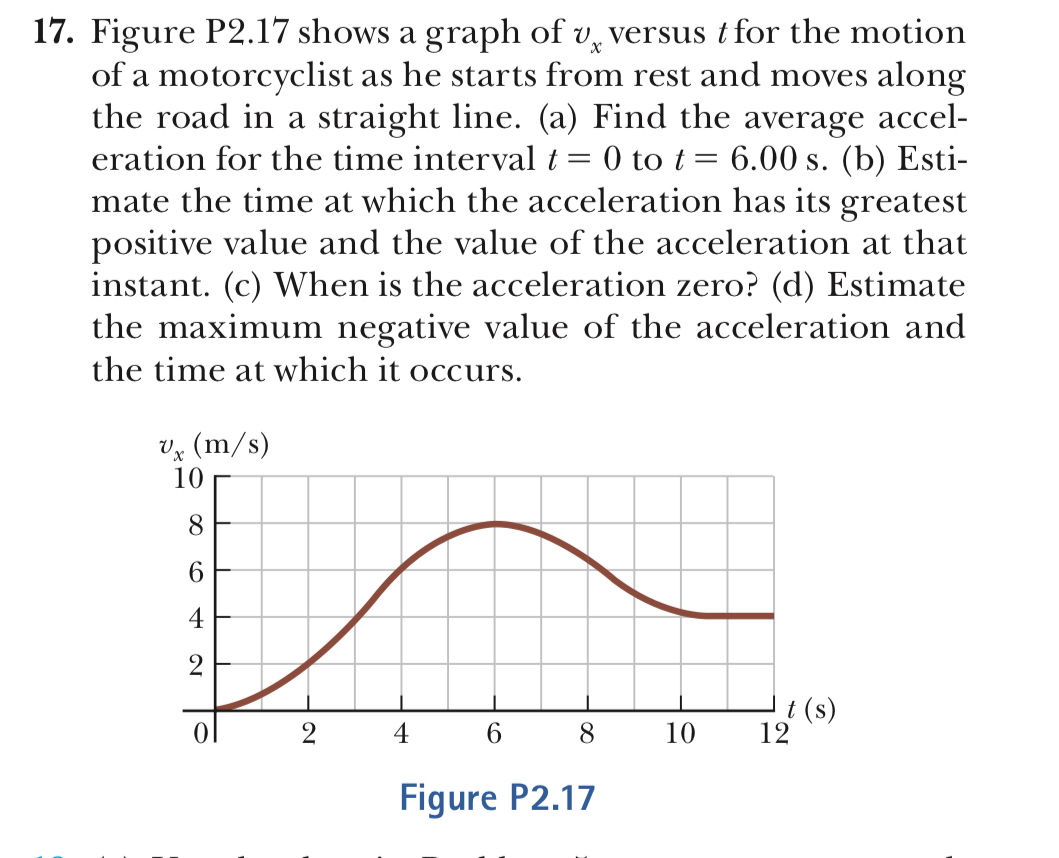# 17. Figure P2.17 shows a graph of v, versus t for the motionof a motorcyclist as he starts from rest and moves alongthe road in a straight line. (a) Find the average acceleration for the time interval0 to t- 6.00 s. (b) Esti-mate the time at which the acceleration has its greatestpositive value and the value of the acceleration at thatinstant. (c) When is the acceleration zero? (d) Estimatethe maximum negative value of the acceleration andthe time at which it occurs.V^ (m/s)1064461012Figure P2.17

Question
180 viewshelp_outlineImage Transcriptionclose17. Figure P2.17 shows a graph of v, versus t for the motion of a motorcyclist as he starts from rest and moves along the road in a straight line. (a) Find the average accel eration for the time interval0 to t- 6.00 s. (b) Esti- mate the time at which the acceleration has its greatest positive value and the value of the acceleration at that instant. (c) When is the acceleration zero? (d) Estimate the maximum negative value of the acceleration and the time at which it occurs. V^ (m/s) 10 6 4 4 6 1012 Figure P2.17 fullscreen
check_circle

Step 1

(a)

The expression for the average acceleration for the time interval t=0 to t=6s,

Step 2

Substitute the values,

Step 3

(b)

The greatest acceleration will occur at that point where the slope has maximum value. SO, the maximum s...

### Want to see the full answer?

See Solution

#### Want to see this answer and more?

Solutions are written by subject experts who are available 24/7. Questions are typically answered within 1 hour.*

See Solution
*Response times may vary by subject and question.
Tagged in

### Physics